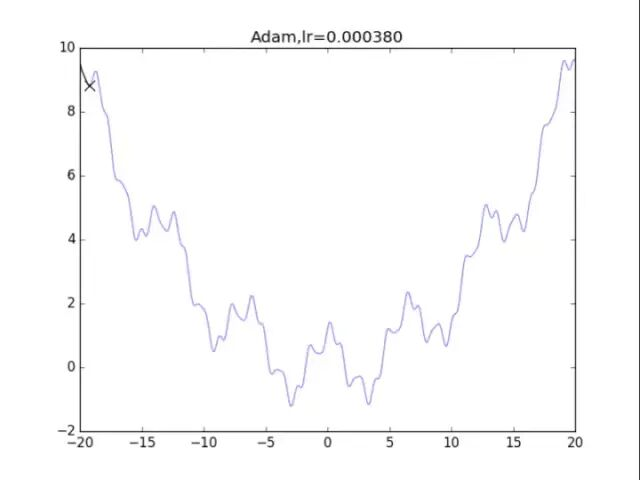帐号 自动登录 找回密码 密码 立即注册

# 深度学习中7种最优化算法的可视化与理解

1、提前遇到局部最小值从而卡住，再也找不到全局最小值了。

2、遇到极为平坦的地方：“平原”，在这里梯度极小，经过多次迭代也无法离开。同理，鞍点也是一样的，在鞍点处，各方向的梯度极小，尽管沿着某一个方向稍微走一下就能离开。

3、“悬崖”，某个方向上参数的梯度可能突然变得奇大无比，在这个地方，梯度可能会造成难以预估的后果，可能让已经收敛的参数突然跑到极远地方去。

## 算法1：纯粹的梯度下降法

` 首先给出学习率lr，初始x `` while True：`` x = x - lr*df/dx`
根据学习率的不同，可以看到不同的效果。学习率过小，卡在局部极小值，学习率过大，压根不收敛。

## 算法2：梯度下降法+动量

算法在纯粹的梯度下降法之上，外加了梯度，从而记录下了历史的梯度情况，从而减轻了卡在局部最小值的危险，在梯度=0的地方仍然会有一定的v剩余，从而在最小值附近摇摆。
` 首先给出学习率lr，动量参数m`` 初始速度v=0,初始x`` while True：`` v = m * v - lr * df/dx`` x += v`
下面可以看图：梯度下降+动量， lr=0.05梯度下降+动量， lr=0.01从中我们可以看出：
1、lr越小越稳定，太大了很难收敛到最小值上，但是太小的话收敛就太慢了。2、动量参数不能太小，0.9以上表现比较好，但是又不能太大，太大了无法停留在最小值处。

缺点是：刚开始参数的梯度一般很大，但是算法在一开始就强力地缩小了梯度的大小，也称学习率的过早过量减少。

算法描述：
`给出学习率lr，delta=1e-7``累计梯度r=0，初始x``while True：`` g = df/dx`` r = r + g*g`` x = x - lr / (delta+ sqrt(r)) * g`效果并不是很好......

## 算法4：RMSProp

算法描述：
`给出学习率lr，delta=1e-6，衰减速率p``累计梯度r=0，初始x``while True：`` g = df/dx`` r = p*r + （1-p）*g*g`` x = x - lr / (delta+ sqrt(r)) * g`RMSProp,p=0.99衰减速率情况复杂，建议自行调参.......

此外还使用了类似于s = s / (1-p1^t)这样的公式，这样的公式在t较为小的时候会成倍增加s，从而让梯度更大，参数跑的更快，迅速接近期望点。而后续t比较大的时候，s = s / (1-p1^t)基本等效于s=s，没什么用。

算法如下：
`给出学习率lr，delta=1e-8，衰减速率p1=0.9，p2=0.999 ``累计梯度r=0，初始x ,一阶矩s=0，二阶矩r=0``时间t = 0``while True：`` t += 1`` g = df/dx `` s = p1*s + (1-p1) *g`` r = p2*r +（1-p2）*g*g`
` s = s / (1-p1^t)`` r = r / (1-p2^t)`
` x = x - lr / (delta+ sqrt(r)) * s`是的，你没有看错，这玩意压根不收敛......表现极差。

在算法中仔细研究后才发现，是在t很小的前几步的时候，p2=0.999太大了，导致r = r / (1-p2^t) 中，1-p2^t接近0，r迅速爆炸，百步之内到了inf。后来修改p2=0.9后效果就好得多了。## 算法6：牛顿法

牛顿法是二阶近似方法的一种，其原理类似于将某函数展开到二次方（二次型）项：### 微信公众号

专注于Qt嵌入式Linux开发等。扫一扫立即关注。### QQ交流群

欢迎加入QQ群大家庭，一起讨论学习！

我有话说......

﻿